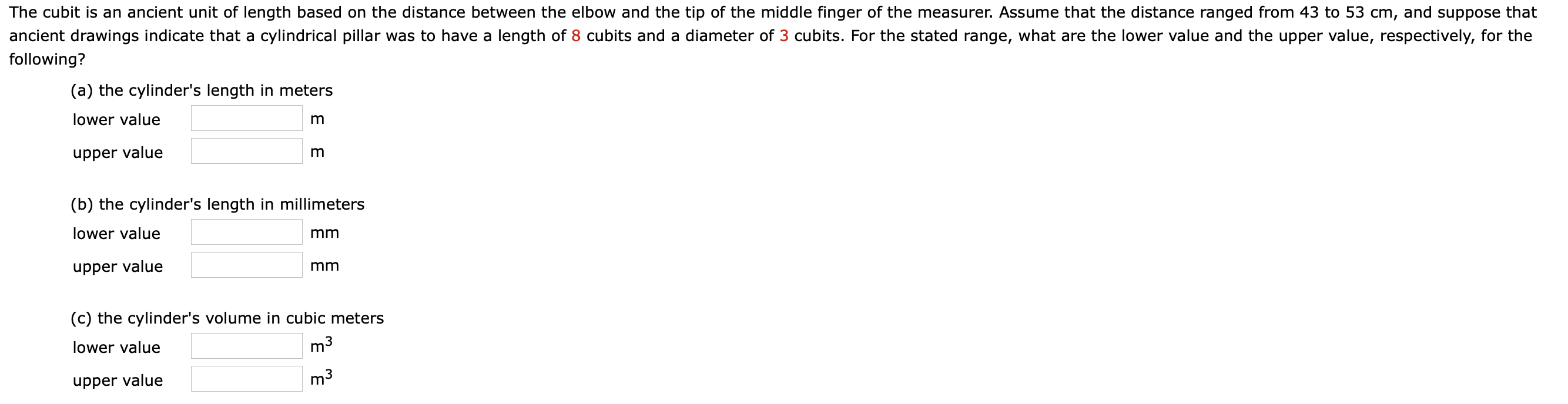# The cubit is an ancient unit of length based on the distance between the elbow and the tip of the middle finger of the measurer. Assume that the distance ranged from 43 to 53 cm, and suppose that ancient drawings indicate that a cylindrical pillar was to have a length of 8 cubits and a diameter of 3 cubits. For the stated range, what are the lower value and the upper value, respectively, for the following? (a) the cylinder's length in meters lower value upper value (b) the cylinder's length in millimeters lower value mm upper value mm (c) the cylinder's volume in cubic meters lower value m3 upper value m3

Question

Help with Chelp_outlineImage TranscriptioncloseThe cubit is an ancient unit of length based on the distance between the elbow and the tip of the middle finger of the measurer. Assume that the distance ranged from 43 to 53 cm, and suppose that ancient drawings indicate that a cylindrical pillar was to have a length of 8 cubits and a diameter of 3 cubits. For the stated range, what are the lower value and the upper value, respectively, for the following? (a) the cylinder's length in meters lower value upper value (b) the cylinder's length in millimeters lower value mm upper value mm (c) the cylinder's volume in cubic meters lower value m3 upper value m3 fullscreen

## Expert Answer

### Want to see this answer and more?

Experts are waiting 24/7 to provide step-by-step solutions in as fast as 30 minutes!*

*Response times vary by subject and question complexity. Median response time is 34 minutes and may be longer for new subjects.
Tagged in
SciencePhysics

### Other© 2021 bartleby. All Rights Reserved.